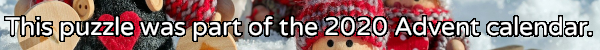mscroggs.co.uk
mscroggs.co.uksubscribe

# Puzzles

## 5 DecemberCarol rolled a large handful of six-sided dice. The total of all the numbers Carol got was 521. After some calculating, Carol worked out that the probability that of her total being 521 was the same as the probability that her total being 200. How many dice did Carol roll?

## Cube multiples

Six different (strictly) positive integers are written on the faces of a cube. The sum of the numbers on any two adjacent faces is a multiple of 6.
What is the smallest possible sum of the six numbers?

## Fair dice

Timothy and Urban are playing a game with two six-sided dice. The dice are unusual: Rather than bearing a number, each face is painted either red or blue.
The two take turns throwing the dice. Timothy wins if the two top faces are the same color, and Urban wins if they're different. Their chances of winning are equal.
The first die has 5 red faces and 1 blue face. What are the colours on the second die?

## Tetrahedral die

When a tetrahedral die is rolled, it will land with a point at the top: there is no upwards face on which the value of the roll can be printed. This is usually solved by printing three numbers on each face and the number which is at the bottom of the face is the value of the roll.
Is it possible to make a tetrahedral die with one number on each face such that the value of the roll can be calculated by adding up the three visible numbers? (the values of the four rolls must be 1, 2, 3 and 4)
Tags: dice

## Archive

Show me a random puzzle
▼ show ▼